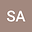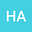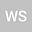loading page

ON COMPUTING LINEARIZING COORDINATES FROM SYMMETRY ALGEBRA
•••• +1
• Sajid Ali,
• Hassan Azad,
• Waqas Shah,
• Fazal Mahomed
Sajid Ali
Zayed University
Author ProfileHassan Azad
Abdus Salam School of Mathematical Sciences
Author ProfileWaqas Shah
Abdus Salam School of Mathematical Sciences
Author ProfileFazal Mahomed
University of the Witwatersrand
Author Profile## Abstract

A characterization of the symmetry algebra of the $N$th-order ordinary differential equations (ODEs) with maximal symmetry and all third-order linearizable ODEs is given. This is used to show that such an algebra $\mathfrak{g}$ determines – up to a point transformation – only one linear equation whose symmetry algebra is $\mathfrak{g}$ and an algorithmic procedure is given to find the linearizing coordinates. The procedure is illustrated by several examples from the literature.